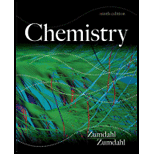# Hydrogen gives off 120. J/g of energy when burned in oxygen, and methane gives off 50. J/g under the same circumstances. If a mixture of 5.0 g hydrogen and 10. g methane is burned, and the heat released is transferred to 50.0 g water at 25.0ºC, what final temperature will be reached by the water?### Chemistry

9th Edition
Steven S. Zumdahl
Publisher: Cengage Learning
ISBN: 9781133611097### Chemistry

9th Edition
Steven S. Zumdahl
Publisher: Cengage Learning
ISBN: 9781133611097

#### Solutions

Chapter
Section
Chapter 6, Problem 58E
Textbook Problem

## Hydrogen gives off 120. J/g of energy when burned in oxygen, and methane gives off 50. J/g under the same circumstances. If a mixture of 5.0 g hydrogen and 10. g methane is burned, and the heat released is transferred to 50.0 g water at 25.0ºC, what final temperature will be reached by the water?

Expert Solution
Interpretation Introduction

Interpretation: The final temperature water mixture to be determined.

Concept Introduction: The heat capacity C is defined as the ratio of heat absorbed to the temperature change. It can be given by,

C = heat absorbedtemperature change

• Require heat of an one gram of substance raise to its temperature by one degree Celsius is called specific heat capacity.

Specific heat capacity = Absorbed heat (J) × Temperature change(c) × mass of substance (g)...(1)

For the above equation heat is:

q = S×M×T.... (1)

q is heat J

M is mass of sample (g)

S is specific heat capacity (J/°C)

T is temperature change ( °C )

For the process no heat loss to the surroundings means then the heat is

(Absorbed) S×M×ΔT=-S×M×ΔT (released)..... (3)

### Explanation of Solution

Explanation

given data:

Energy given by hydrogen is 120. J/g

Energy given by methane is 120. J/g

Mass of water is 50.0 g

Initial temperature of water is 25.0 °C

Specific heat capacity of water is 4.18J /°C.g .

To determine: Final temperature of the water.

Energy given by hydrogen (qH) + Energy given by methane (qmethane) = Energy gained by water   (qw)...... (4)

5.0 g ×120 J/g H+10 g×50 J/CH4 g = 1100 J                                             1100 J = 4.18J /C.g×50

### Want to see the full answer?

Check out a sample textbook solution.See solution

### Want to see this answer and more?

Bartleby provides explanations to thousands of textbook problems written by our experts, many with advanced degrees!

See solution